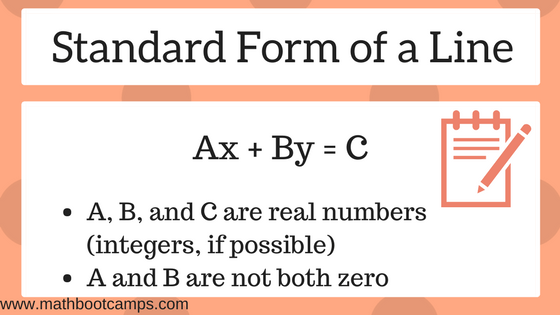# Standard form of a line (with examples)

The standard form of a line is simply a special way of writing the equation of a line. You are probably already familiar with the slope-intercept form of a line, y = mx + b. The standard form is just another way to write this equation, and is defined as Ax + By = C, where A, B, and C are real numbers, and A and B are both not zero (see note below about other requirements). As you will see in the lesson below, every line can be expressed in this form.

Note that the requirements on A, B, and C vary a bit from textbook to textbook. While they technically can be any real numbers, it is most common to write them as integers and to keep A positive. It is also common to require that A, B, and C share no common factors. We will follow these rules in this lesson.

## Finding the standard form of a line

We will now look at some examples of writing the standard form of a line, when the line is already given in y = mx + b form.### Example

Write the equation of the line in standard form:
$$y = -2x + 4$$

### Solution

This is the easiest case, because the coefficients on x and y are already integers (negative or positive whole numbers). This means that we just need to bring the x-term to the other side.

$$y = -2x + 4$$

$$2x + y = 4$$

The standard form of the line is:
$$\bbox[border: 1px solid black; padding: 2px;]{2x + y = 4}$$

A more complicated case is when either the slope, the constant, or maybe even both are fractions. Since the coefficients on x and y are preferably written as integers, this means that you have to “clear fractions”. This is shown in the next example.

### Example

Write the equation of the line in standard form.
$$y = -\dfrac{2}{3}x + \dfrac{1}{4}$$

To clear fractions, you can multiply both sides of the equation by a whole number. The whole number should be a multiple of both denominators (ideally it is the least common multiple). Our denominators here are 3 and 4, so we will use 12.

Multiply both sides by 12 to clear fractions.

\begin{align}y &= -\dfrac{2}{3}x + \dfrac{1}{4}\\ 12(y) &= 12\left(-\dfrac{2}{3}x + \dfrac{1}{4}\right)\\ 12y &= -\dfrac{24}{3}x + \dfrac{12}{4}\\ 12y &= -8x + 3\end{align}

Now we can add 8x to both sides to get the standard form of the line.

$$\bbox[border: 1px solid black; padding: 2px]{8x + 12y = 3}$$

## Finding the slope from the standard form of a line

You can always find the slope by solving for y and rewriting the equation in slope-intercept form y = mx + b. However, it can be shown that when the equation of a line is written in standard form, the slope is always $$–\dfrac{A}{B}$$. This quick calculation can allow you to find the slope of any line written in this form without needing to apply extra algebra steps.

### Example

What is the slope of the line defined by the equation below?
$$5x + 8y = -2$$

### Solution

The slope is $$–\dfrac{A}{B}$$, and here A = 5 and B = 8. Therefore:

\begin{align}\text{slope} = m &= -\dfrac{A}{B}\\ &= \bbox[border: 1px solid black; padding: 2px]{-\dfrac{5}{8}}\end{align}